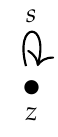Matthew wrote:

> Hopefully someone can come along and say definitively what the objects are in the category of endofunctors of a category \$$\mathcal{C}\$$.

The objects of the endofunctor category of \$$\mathcal{C}\$$ are functors \$$F : \mathcal{C} \to \mathcal{C}\$$. The morphisms are natural transformations between such functors.

This is a special case of something we'll talk about soon. Given two categories \$$\mathcal{C}, \mathcal{D}\$$, the **functor category** \$$\mathcal{D}^\mathcal{C}\$$ has:

* functors \$$F: \mathcal{C} \to \mathcal{D}\$$ as objects,
* natural transformations between such functors as morphisms.

In this notation the endofunctor category of \$$\mathcal{C}\$$ is \$$\mathcal{C}^\mathcal{C}\$$.

We haven't talked about natural transformations in this course yet, so the following puzzles are "unfair", but I can't resist mentioning them. Maybe I'll make them official puzzles later.**Puzzle.** If \$$\mathbf{N}\$$ is the free category on the graphwhat is the endofunctor category \$$\mathbf{N}^\mathbf{N}\$$?

**Puzzle.** What is the functor category

$\mathbf{N}^{(\mathbf{N}^\mathbf{N})} ?$

We could go on...